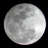# OnBalanceVolume (OBV) with Moving Averages for ThinkorSwim#### BenTen

Staff
VIP
This indicator consists of the On Balance Volume (OBV) indicator along with two custom moving averages of the standard OBV. It was shared by Ken Rose (@KRose_TDA).### thinkScript Code

Code:
``````#Follow @KRose_TDA on twitter for updates to this and other scripts
#this script provides 2 moving averages of the study chosen
#default study in OnBalanceVolume Volume
declare lower;
#place the study desired after reference in the line below you must include the brackets "()"
#Initial study is OnBalanceVolume
plot MyStudy = reference OnBalanceVolume();
MyStudy.SetDefaultColor(GetColor(4));

input Moving_Averge_One_Length = 20;
input Moving_Averge_Two_Length = 50;
Input Line_Weight_MyStudy = 4;
Input Line_Weight_Moving_Average_One = 3;
Input Line_Weight_Moving_Average_Two = 3;
MyStudy.setlineWeight(Line_Weight_MyStudy);
plot MaOne = Average(mystudy, Moving_Averge_One_Length);
MaOne.SetDefaultColor(GetColor(6));
MaOne.setlineWeight(Line_Weight_Moving_Average_One);
Plot MaTwo = Average(mystudy, Moving_Averge_Two_Length);
MaTwo.SetDefaultColor(GetColor(8));
MaTwo.setlineWeight(Line_Weight_Moving_Average_Two);``````

https://tos.mx/nLH4MUi
Before using this, be sure to watch the following video, so you get an understanding of how it works.

•OBV Histogram with Paintbars for ThinkorSwim Indicators 13